[BACK]Computer Modeling in Engineering & SciencesDOI: 10.32604/cmes.2022.019440

ARTICLE

Stability Analysis of Predator-Prey System with Consuming Resource and Disease in Predator Species

1Department of Mathematics, Air University, PAF Complex E-9, Islamabad, 44000, Pakistan
2Department of Mathematics, King Abdulaziz University, Jeddah, 22254, Saudi Arabia
3Department of Mathematics and Sciences, College of Humanities and Sciences, Prince Sultan University, Riyadh, 11586, Saudi Arabia
*Corresponding Author: Muhammad Shoaib Arif. Email: marif@psu.edu.sa
Received: 24 September 2021; Accepted: 13 January 2022

Abstract: The present study is concerned with formulating a predator-prey eco-epidemiological mathematical model assuming that an infection exists in the predator species. The two classes of predator species (susceptible and infected) compete for the same sources available in the environment with the predation option. It is assumed that the disease does not spread vertically. The proposed model is analyzed for the stability of the coexistence of the predators and prey. The fixed points are carried out, and the coexisting fixed point is studied in detail by constructing the Lyapunov function. The movement of species in search of food or protection in their habitat has a significant influence, examined through diffusion. The ecological influences of self-diffusion on the population density of both species are studied. It is theoretically proved that all the under consideration species can coexist in the same environment. The coexistence fixed point is discussed for both diffusive and non-diffusive cases. Moreover, a numerical scheme is constructed for solving time-dependent partial differential equations. The stability of the scheme is given, and it is applied for solving presently modified eco-epidemiological mathematical model with and without diffusion. The comparison of the constructed scheme with two exiting schemes, Backward in Time and Central in Space (BTCS) and Crank Nicolson, is also given in the form of plots. Finally, we run a computer simulation to determine the effectiveness of the proposed numerical scheme. For readers’ convenience, a computational code for the proposed discrete model scheme may be made available upon request.

Keywords: Eco-epidemiology; self-diffusion; stability; Lyapunov function; proposed numerical scheme

1  Introduction

The pronounced work of Lotka et al. [1,2] in the field of predation prey relationship studies has made this area significant for research purposes. Great attention by scientific society has brought this field to the next level, as evident in population dynamics and mathematical ecology. The pioneering work of Kermack and McKendrick  has opened a new gospel in epidemiology. Following the work of , Maiti et al.  have studied the ratio-dependent predator-prey model and explored this model’s stochastic and deterministic features . Peculiarly observed four variable balancing points and static factors of the system to understand how the delay affects the system’s movements. Moreover, Hopf bifurcation  is discussed by using bifurcation theory.

Wang et al. constructed a model whose stages were constructed on predation prey strategy to study a delay, stage-structured model. This study showed the delay when the prey population was at its immature stage and drew some essential ecological conclusions by analyzing the model in terms of global stability. Considering the study made by Zang et al. [6,7] and his coworkers examined the eco-epidemiological model and alleged that sickness is spreading among prey populations. This study took standard incidents and observed the behavior of the proposed system in all potential equilibrium to formulate conclusions. Additionally, Haque and coworkers examined Hopf bifurcation theory. Following Holling-Tanner functional response, Haque and venturino did a predation prey study; however, in , Yi et al. have investigated the concept of control and configurability in the context of predation prey paradigm of behavior. Some authors have discussed the chaotic and non-chaotic behavior of the dynamical system and presented optimal chaos control to overcome chaotic behavior . Hyper chaotic behavior of the non-autonomous cardiac conduction system and overcome techniques are discussed in . Some authors investigated the dynamical behavior of tri-atomic molecules using the fractional calculus approach .

Ecology and epidemiology are two distinct fields with fundamental effects on scientific work and society. However, there are numerous parallels between the two fields. Nowadays, scientists have merged both fields to form a new area known as eco-epidemiology that has become increasingly important, as indicated in the studies . Proposes and analyses a predator-prey system where predators infect prey due to the polluted environment’s influence . Sinha et al.  discovered that diseased prey is more venerable than predators living in a polluted environment. Many writers in the literature suggested eco-epidemiological models that focused solely on disease in the target population . A Lotka-Volterra type predator-prey system was examined by Johri and colleagues in which an illness infected the prey. They investigated the local and global stability and its plausibility; however, Mukhopadhyaya et al.  investigated how diffusion and delay affected the system’s dynamics by considering a prey-predator model with Holling type II functional response. Following their efforts, Greenhalgh et al.  formulated the influence of diffusivity on the stability and persistence focused discussion of the model using a predator-prey model with disease in the prey population. To compare with Hu et al.  three-dimensional prey-predator delayed model, Chattopadhyay et al.  created highly organized two and three-dimensional models that articulated the local stability results and compared their findings to Hu and Li’s findings. A fractional chaotic system with order two and three nonlinearities is discussed in ; moreover, NSFD is suggested for the model. A fractional predator-prey model is investigated in  using some uncertain initial conditions, and the authenticity of the proposed model is proved via numerical simulation. In , the authors investigated the two-strain epidemic model. They have analyzed the model having two vaccinations. Liu et al.  analyzed semi-trivial, infection-free, and predator-free equilibrium situations using the impulsive predator-prey model; however, Naji et al. [25,26] examined eco-epidemiology’s local and global features of the delayed autonomous predator-prey model. Xiao et al.  has introduced the application of partial differential equation using the predator-prey model with infected prey population known as “prey infection system.” The researcher realizes conversing prey to predator is constant (i.e., independent of gestation period) if k=k0ed(τ)¯ Where dτ¯ are mortality and delay, respectively. Xiao et al.  Elaborated that the delay could have a stable and DE stable effect on the system, narrating the positive and negative equilibrium. Linear models for series RL and RC are studied using truncated M-derivative operators . Local asymptotic stability is discussed for transmission dynamics of SARS-Cov-2 . Numerical simulations are provided using RK-2, RK-4, and Euler methods. Some authors used the technique of optimal control to investigate the stochastic and deterministic Hepatitis B Virus model .

Additional work on ecology and epidemiology can be seen in . Musa et al.  examined the predator-prey system in which the predator population was infected with the pathogen. Their findings showed that infection among predators acts as a counterbalance to the predator of enrichment, and parasites removing themselves from food webs could have disastrous penalties. Biological control and resource management of these parasites were also part of their research. Haque  Proposed that the introduction of the pathogen in the predatory population acts as a biological control that maintains the predator’s population. Pal et al.  investigated a model in which predators were infected with the pathogen and determined their instability; however, several studies were based on the pathogenicity of both predator and prey, focusing on local or global equilibrium. Pattern formation like Turing spots and spiral-like structures in the predator-prey system are discussed . Han et al. , using SIS or SIR, proposed four modifications to the prey-predator model, and the threshold of alterations caused by parasite infections was studied. They looked at models with both standard and mass action incidences, as well as a combination of the two. The global stability for the COVID-19 model is discussed in detail in , using actual data of Pakistan. Caputo type differential operator is employed to analyze the linear mass springer damper system . Hsieh et al.  introduced a model that examined the pathogenicity of both predator and prey hence measuring a large number of threshold parameters for local analysis of distinct equilibria of the proposed system. They confer that the different combinations of variables may lead the infection to be eradicated from the environment due to the killing of infected prey. Hence, they demonstrated that illness in both populations could either promote or impede coexistence. The precise influence of infection on coexistence must be determined in each situation. After this work is completed, it will be able to propose more applications for the current methodology . Some researchers have provided the numerical solution of the predator-prey model through numerical schemes like Adomian Decomposition, El Kalla, etc. The comparison between exact and numerical solutions is also provided in plots . Qureshi et al.  studied the blood ethanol, concentration model. For fractionalizing the model, three different fractional operators are used.

From the available literature, it is found that some authors have discussed in detail the stability of the predator-prey system with common food resources . The authors have considered the stability of the diffusive and kinetic system. We got the main motivation from the literature for the present work to the best of our knowledge. Nobody has discussed the problem of multi-species coexistence, keeping in view the common food resources and infection in one of the two species (predator). Therefore, we formulated a predator-prey model with a common food resource for both the predator and prey under the assumption that a fatal disease exists in the predator. We have proved by constructing the Lyapunov function that for this complex system, both the species predator (Susceptible and Infected) and prey can coexist in the ecosystem with and without diffusion.

Moreover, we have constructed a third-order numerical scheme for the model, and a faster scheme convergence than BTCS and Crank Nicolson is obtained. To the best of our knowledge, such a system considering disease and common food resources is not much discussed in the literature. We are hopeful that this particular research has an attraction for the eco-epidemiologists because they can better understand the complex behavior of species in the ecosystem by looking at the stability part in Sections 3 and 4. This particular study focuses on the coexistence of both species in the presence of disease in the predator for both diffusive and non-diffusive cases.

The present investigation provides a multi-species eco-epidemiological model discussing the existence of all species, which is the key result in such models. The findings in the present study show the survival of all the species in the same habitat. Some phase portraits are presented to show the behavior of species. Additionally, a faster convergence scheme is developed, which gives better results as compared to some existing schemes. The proposed scheme can help study such models.

The current study focuses on the formulation and analysis of the epidemiological predator-prey model in the sequence described here. Section 2 deals with the formulation of the multi-species model under the assumption that predator species have some fatal disease that can spread from predator to predator only. Moreover, the two classes of predators compete for the common food resources and the predation option. Sections 3 and 4 deal with the stability of the model for both non-diffusive and diffusive cases, respectively. For the sake of simulations, we constructed a third-order numerical scheme for the solution of the model in Section 5. The stability of the proposed scheme is also proved in the same section using the Von Neumann stability technique. In Section 6, numerical simulations are performed, and a comparison of the constructed scheme with Backward in Time and Central in Space and Crank Nicolson is displayed in the form of plots. Section 7 presents the discussion on the plots and findings of the present study, whereas the conclusion is provided in Section 8. Finally, some future directions are suggested in the last part of this research.

2  Model Formulation

The ecosystem is a complex natural structure where interacting species affect the whole environment and the existing species. Many species depend on the same resources, which gives rise to competition among them. Moreover, the interacting species are at the danger of infectious diseases in the real environment. Many researchers presented mathematical models to discuss the effects of infection on population density. The infections are so critical that they disturb the whole ecosystem. This problem motivated several investigators to put their model to study the population dynamics effectively. Here we propose a model to study the predator-prey system. The assumption of the models are as under:

H1: The predator species have two classes; susceptible and infected class.

H2: The disease can only spread from predator to predator, and the disease is mortal.

H3: The transmission is non-vertical.

H4: The predator classes depend on the same food resource f with different consumption rates a and b, respectively; additionally, both classes have predation activity as well.

In the light of the above assumptions, we take US(t),UI(t) as the predators (susceptible and infected) population density and V(t) as prey population density at any time t. Here we assume that U=US+UI is the total population density of predator species.

The above assumptions lead to the following model:

dUS(t)dt=US(afaUS+bUI+α1VβUIm1),t>0(1)

dUI(t)dt=UI(bfaUS+bUI+α2V+βUS(µ+m1)),t>0(2)

dV(t)dt=V(cgα1USα2UIn1),t>0(3)

where

Φ0={US(0)=US,00,UI(0)=UI,00,V(0)=V00}(4)

is the set of initial conditions. Here m1 and n1 are the natural mortality rates of the predator and prey population, respectively, whereas µ is the death rate of an infected predator due to disease, f stands for the same food resources for both predator classes; and a,b are the consumption rates for susceptible and infected predators. The parameters α1 and α2 respectively represent the predations rates for both predator classes, whereas β stands for the transmission rate of infection from infected predators to susceptible predators. The parameter g displays the food resources for the prey population, and c is the consumption rate. Moreover, due to infection, the mortality rate of infected predators is high as compared to the susceptible class.

We can rewrite the above system of equations as

dUSdt=US(afaUS+bUI+α1VβUIm1),t>0(5)

dUIdt=UI(bfaUS+bUI+α2V+βUSm2),t>0(6)

dVdt=V(cgα1USα2UIn1),t>0(7)

Here the parameter m2 stands for the death rate of infected predators. It is obvious from the above discussion that m2 > m1.

3  Stability for the Predator-Prey System of Equations

In the present section, we compute the equilibria of the system. The equilibrium points are simply the solutions of the following system of equations:

0=US(afaUS+bUI+α1VβUIm1)(8)

0=UI(bfaUS+bUI+α2V+βUSm2)(9)

0=V(cgα1USα2UIn1)(10)

One can solve the above system of equations to find the equilibrium points which are

E1=(0,fm2,0),E2=(fm1,0,0),E3=(US,3,UI,3,V3)

E4=(US,4,UI,4,V4),E5=(US,5,UI,5,V5),E6=(US,UI,V)

where

US,3=0,UI,3=cgn1α2,V3=cgm2fα2n1m2α2(cgn1)

US,4=am22bfβbm1m2β(am2bm1),UI,4=afβam1m2+bm2β(am2bm1),V4=0

US,5=cgn1α1,UI,5=0,V5=cgm1fα1n1m1α1(cgn)

US=((α1f+m1(cgn1)α2(cgn1)(α1m1β(cgn1)))bafα22(aα2bα1)(α2m1+m2α1β(cgn1))(11)

UI=((α2f+m2(cgn1)α1(cgn1)(α2m1+β(cgn1)))abfα12(aα2bα1)(α2m1+m2α1β(cgn1))(12)

V=((b(cgn1)m1a(cgn1)m2+f(aα2bα1)β+(α2m1+m2α1)(bm1+m2a)(aα2bα1)(α2m1+m2α1β(cgn1))(13)

In the present section, we study the stability of the fixed point, which deals with the coexistence of all the species. The existence condition for equilibrium point is

ϕ={(US,UI,V),US>0,UI>0,V>0}(14)

Theorem 1: Let a,b and f be positive and (11) holds then (US,UI,V) of (5)(7) is stable in

ω={(US,UI,V),US>0,UI>0,V>0}

Proof: We construct the Lyapunov function to determine the stability of the coexistence equilibrium point (US,UI,V). Consider the following:

L(US,UI,V)=USUSηUSηdη+UIUIκUIκdκ+VVξVξdξ(15)

By taking derivative with respect to “t”, we have

dLdt=USUSUSdUSdt+UIUIUIdUIdt+VVVdVdt

dLdt=(USUS)(afaUS+bUI+α1VβUIm1)+(UIUI)(bfaUS+bUI+α2V+βUSm2)+(VV)(cgα1USα2UIn1)

After some simplification, we get

dLdt=(USUS)afaUS+bUIaUSbUI(aUS+bUI)(aUS+bUI)+(UIUI)bfaUS+bUIaUSbUI(aUS+bUI)(aUS+bUI)

dLdt=f(aUS+bUI(aUS+bUI))2(aUS+bUI)(aUS+bUI)0(16)

The above result proves the Lyapunov stability for the system of Eqs. (5)(7).

4  Stability for Diffusive System

The dynamical population model, random walk influences the structure of the habitat. This movement can be varied from one place to the other depending on the factors like food resources and protection in the environment. In addition, species can vary with respect to density in the area they live. The following section presents the effect of diffusion on the coexistence of species.

4.1 Global Stability for the Self-Diffusive System

The self-diffusion system for (5)(7) can be written as

dUS(x,t)dt=d11US+US(afaUS+bUI+α1VβUIm1),xϵΩ,t>0(17)

dUI(x,t)dt=d22UI+UI(bfaUS+bUI+α2V+βUSm2),xϵΩ,t>0,(18)

dV(x,t)dt=d33V+V(cgα1USα2UIn1),xϵΩ,t>0(19)

where USϑ=UIϑ=Vϑ=0 , xϵΩ,t>0

Here US(x,t), UI(x,t) and V(x,t) respectively represent the population density of susceptible predator, infected predator, and prey at location x ϵ Ω and time t. Here Ω represents the bounded domain having smooth boundary Ω, and ϑ is the outward unit normal on the smooth boundary. The Neumann boundary conditions show the zero population flux across the boundary.

The initial condition is as under

Φ0={US(x,0)=US,0(x)0,UI(x,0)=UI,0(x)0,V(x,0)=V0(x)0}(20)

Here d11,d22,d33 are non-negative self-diffusion parameters. According to Hollis results, the solution to the above system has global existence and boundedness . The self-diffusive system has an equilibrium point E6=(US,UI,V) under the assumption (11).

Theorem 2 Let Ω be bounded domain with smooth boundary Ω. Suppose a,b,d11,d22,d33 and f be positive and (11) holds then (US,UI,V) of (14)(16) is stable in

ω={(US,UI,V),US>0,UI>0,V>0}

Proof. It is obvious that {(US,UI,V),US=0}, {(US,UI,V),UI=0} and {(US,UI,V),V=0} are the invariant manifolds. We establish the Lyapunov function to determine the global stability for the self-diffusive system as under

L(U,VS,VI)=ΩUSUSηUSηdηdX+ΩUIUIκUIκdκdX+ΩVVξVξdξdX(21)

Now taking derivative with respect to “t” on both sides, we get

dLdt=ΩUSUSUSUSdtdX+ΩUIUIUIUIdtdX+ΩVVVVdtdX

=Ω(USUSUS)(d11US+US(afaUS+bUI+α1VβUIm1))

+Ω(UIUIUI)(d22UI+UI(bfaUS+bUI+α2V+βUSm2))

+Ω(VVV)(d33V+V(cgα1USα2UIn1),

=M1+M2+M3+M4(22)

where

M1=d11USΩ1US2|US2|dX0(23)

M2=d22UIΩ1UI2|UI2|dX0(24)

M3=d33VΩ1V2|V2|dX0(25)

M4=Ω(USUSUS)[US(afaUS+bUI+α1VβUIm1)]dX+Ω(UIUIUI)[UI(bfaUS+bUI+α2V+βUSm2)]dX+Ω(VVV)[V(cgα1USα2UIn1)]dX0(26)

M4=Ωf(aUS+bUI(aUS+bUI))2(aUS+bUI)(aUS+bUI)0(27)

From

dLdt0(28)

Hence (US,UI,V) is globally stable.

5  Construction of Numerical Scheme

An implicit scheme on three-time levels is constructed. The construction of the scheme is started with the difference equation given as

uin+1=auin+uin14+Δt{b(ut)in+1+c(ut)in+e(ut)in1}(29)

The Taylor series expansions for uin+1,uin1,(ut)in+1 and (ut)in1 are given as

uin+1=uin+Δt(ut)in+(Δt)22(2ut2)in+(Δt)36(3ut3)in+O((Δt)4)(30)

uin1=uinΔt(ut)in+(Δt)22(2ut2)in(Δt)36(3ut3)in+O((Δt)4)(31)

(ut)in+1=(ut)in+Δt(2ut2)in+(Δt)22(3ut3)in+O((Δt)3)(32)

(ut)in1=(ut)inΔt(2ut2)in+(Δt)22(3ut3)in+O((Δt)3)(33)

Substituting Taylor series expansions (30)(33) into Eq. (29), we obtain the following:

uin+Δt(ut)in+(Δt)22(2ut2)in+(Δt)36(3ut3)in+O((Δt)4)=auIn+14(uinΔt(ut)in+(Δt)22(2ut2)in(Δt)36(3ut3)in+O((Δt)4)+Δt{b(ut)in+bΔt(2ut2)in+b(Δt)22(3ut3)in+c(ut)in+e((ut)inΔt(2ut2)in+(Δt)22(3ut3)in)}(34)

Comparison of coefficients of uin,Δt(ut)in,(Δt)2(2ut2)in and (Δt)3(3ut3)in on both sides of Eq. (34) leads to the following:

1=a+14(35)

1=14+b+c+e(36)

12=18+be(37)

16=124+b2+e2(38)

Solving Eqs. (35)(38) gives the values of an unknown parameters a,b,c and e as

a=34,b=1948,c=56,e=148(39)

5.1 Stability Analysis

The stability of the numerical scheme for the diffusive system is checked by applying the Von Neumann stability criterion. For this criterion, the difference equation obtained by applying a numerical scheme is transformed to another equation containing amplitudes and phase angle of the waves. After that, stability condition is imposed on the obtained equation, which is based on the quotient of amplitudes of the wave at (n+1)th'' and nth levels. For starting the stability procedure, consider the following diffusive linear predator-prey equation having the form:

ut=d12u2x+α¯u(40)

The discretization of Eq. (40) using the presented scheme is given as

uin+1=auin+14uin1+Δt{bd1(ui+1n+12uin+1+ui1n+1(Δx)2)+bα¯uin+1+cd1(ui+1n2uin+ui1n(Δx)2+α¯uin)+cα¯uin+ed1(ui+1n12uin1+ui1n1(Δx)2+eα¯uin1)}(41)

According to Von Neumann stability criterion, consider the following transformations:

uin+1=En+1eiIψ,ui±1n=Ene(i±1)Iψ,ui±1n1=En+1e(i±1)Iψ where I=1(42)

Substituting transformations (42) into Eq. (41), the following is obtained:

En+1eiIψ=aEneiIψ+14En1eiIψ+Δt{bd1(e(i+1)Iψ2eiIψ+e(i1)Iψ(Δx)2)En+1+α¯En+1eiIψ+cd1(e(i+1)Iψ2eiIψ+e(i1)Iψ(Δx)2)En+cα¯EneiIψ+ed1(e(i+1)Iψ2eiIψ+e(i1)Iψ(Δx)2)En1+eα¯En1eiIψ}(43)

Dividing both sides of Eq. (43) by eiIψ yields

En+1=aEn+14En1+Δt{bd1(2cosψ2(Δx)2)En+1+α¯En+1+cd1(2cosψ2(Δx)2)En+cα¯En+ed1(2cosψ2(Δx)2)En1+eα¯En1}(44)

Collecting coefficients of En+1 on the left-hand side of Eq. (44) provides

(12dd1Δtα¯)En+1=aEn+14En1+2cdd1(cosψ1)En+Δtα¯En+1+2edd1(cosψ1)En1+eΔtα¯En1(45)

where d=Δt(Δx)2.

Eq. (45) can be expressed as

En+1=AEn+BEn1(46)

where

A=a+2cdd1(cosψ1)+cΔtα¯12dd1Δtα¯ and B=b+2edd1(cosψ1)+eΔtα12dd1Δtα¯(47)

Since the presented numerical scheme is constructed on three-time levels, one more equation is required to get an amplification matrix. An additional equation is expressed as

En=1.En+0.En1(48)

System of Eqs. (46) & (48) can be expressed in the following matrix-vector equation:

[En+1En]=[AB10][EnEn1](49)

The amplification factor is a matrix, and the stability conditions can be imposed on the Eigenvalue of the amplification matrix and these conditions are expressed as

|A+A2+4B2|1and|AA2+4B2|1(50)

The scheme will be stable if it satisfies inequalities (50) for positive eigenvalues of the amplification matrix, and if the eigenvalues of an amplification matrix are negative, then the stability condition is expressed as

|B|21(51)

where A & B are expressed in (47).

6  Numerical Simulation

The presented third-order multistep finite difference scheme can efficiently be applied for solving the eco-epidemiological population model. Here, this scheme will be applied for the numerical simulation of the model presented in (17)(19). The model presentation under the scheme applied is given as

US,in+1=aUS,in+14US,in1+Δt[b{d11(US,i+1n+12US,in+1+US,i1n+1)/(Δx)2+US,in+1(afaUS,in+1+bUI,in+1+α1Vin+1βUI,in+1m1)}+c{d11(US,i+1n2US,in+US,i1n)/(Δx)2+US,in(afaUS,in+bUI,in+α1VinβUI,inm1)}+e{d11(US,i+1n12US,in1+US,i1n1)/(Δx)2+US,in1(afaUS,in1+bUI,in1+α1Vin1βUI,in1m1)}](52)

UI,in+1=aUI,in+14UI,in1+Δt[b{d22(UI,i+1n+12UI,in+1+UI,i1n+1)/(Δx)2+UI,in+1(bfaUS,in+1+bUI,in+1+α2Vin+1+βUS,in+1m2)}+c{d22(UI,i+1n2UI,in+UI,i1n)/(Δx)2+UI,in(bfaUS,in+bUI,in+α2Vin+βUS,inm2)}+e{d22(UI,i+1n12UI,in1+UI,i1n1)/(Δx)2+UI,in1(bfaUS,in1+bUI,in1+α2Vin1+βUS,in1m2)}](53)

Vin+1=aVin+14Vin1+Δt[b{d33(Vi+1n+12Vin+1+Vi1n+1)/(Δx)2+Vin+1(cgα1US,in+1α2UI,in+1n1)}+c{d33(Vi+1n2Vin+Vi1n)/(Δx)2+Vin(cgα1US,inα2UI,inn1)}+e{d33(Vi+1n12Vin1+Vi1n1)/(Δx)2+Vin1(cgα1US,in1α2UI,in1n1)}](54)

The above Eqs. (52)(54) give the discretization of the diffusive system.

7  Discussion

We presented an eco-epidemiological model for the predator-prey system in the present study. Two classes of predator species (susceptible and infected) with disease transmission from predator to predator are considered. The transmission of infection is supposed to be non-vertical in the present research. We have proved the coexistence of predator and prey in the same habitat. Figs. 1 & 2 show the convergence of three numerical schemes for the modified model of the eco-epidemiological diffusive mathematical model using different parameter values. It is clear from the plot that faster convergence is obtained using the proposed numerical scheme. The maximum number of norms for each Eqs. (52)(54) is determined over the number of iterations used by each scheme, and Figs. 1 & 2 illustrate the convergence speed. Since the proposed scheme is third-order accurate, it converges faster than existing first and second-order schemes in time. It is to be noted that the second-order central space discretization is used for three schemes. Figs. 3 & 4 demonstrate the comparison of solutions obtained by utilizing three numerical schemes over time considering two different data sets, while Figs. 5 & 6 show the comparison of schemes over the spatial coordinate x. The phase portraits for the ODEs model are given in Figs. 79 that express the relationships between Us,UI & V. The initial conditions for drawing phase portraits are obtained in the interval (0,1). In Figs. 1 & 2, Nx,Nt, tf & L respectively show the number of grid points, the number of time levels, final time, and length of the domain.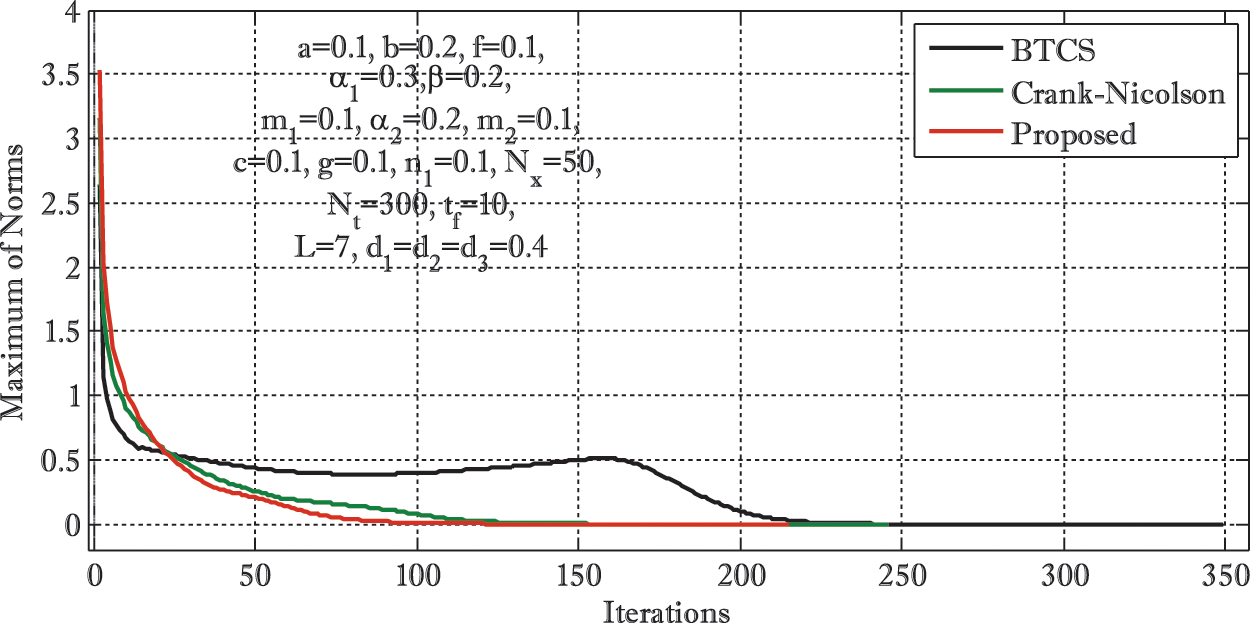Figure 1: Convergence of three schemes using a=0.1,b=0.2,f=0.1,α1=0.3,β=0.2,m1=0.1,α2=0.2,m2=0.1,c=0.1,g=0.1,n1=0.1,Nx=50,Nt=300,tf=10,L=7,d1=d2=d3=d4=0.4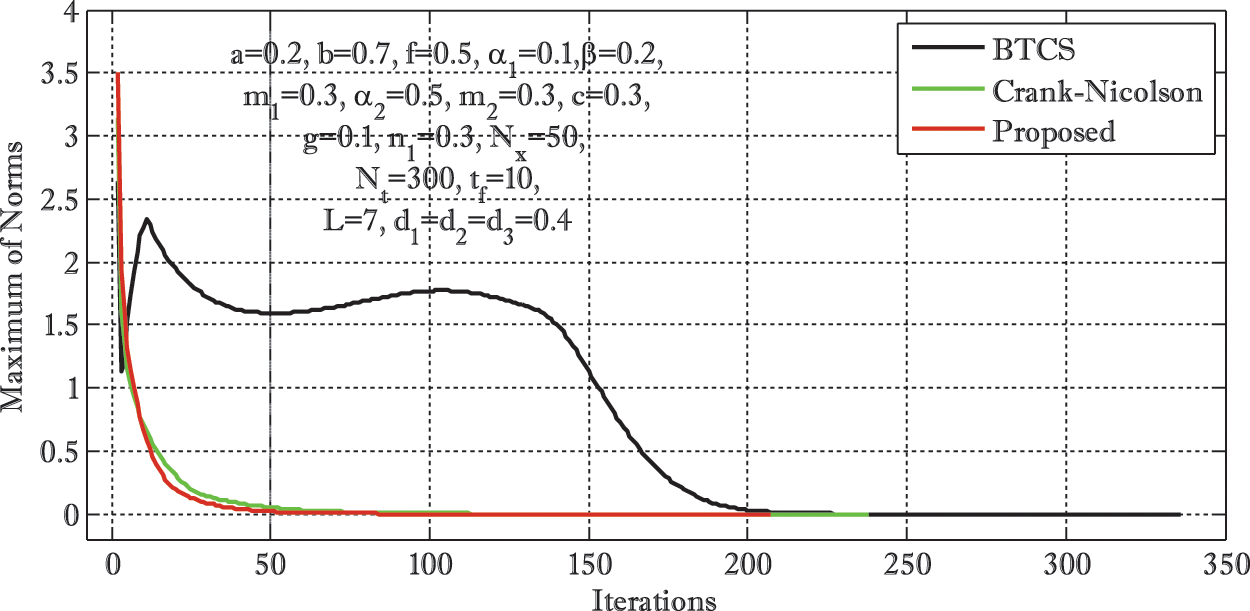Figure 2: Convergence of three schemes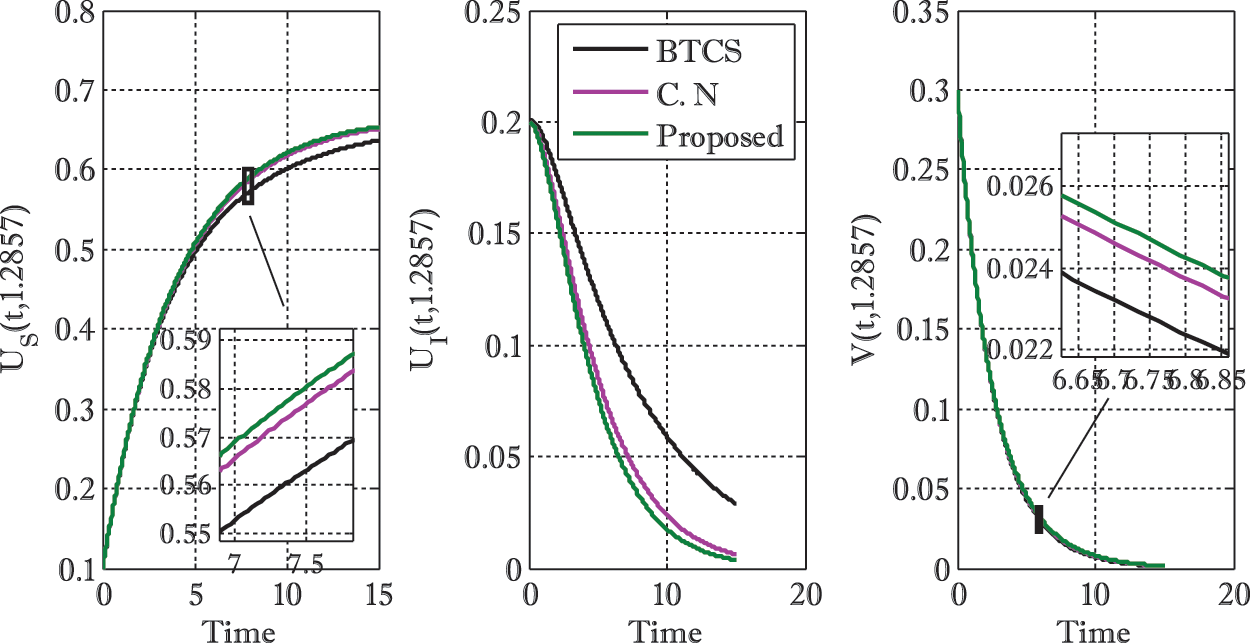Figure 3: Comparison of three schemes over time using a=0.1,b=0.01,f=0.2,α1=0.1,β=0.2,m1=0.3,α2=0.5,m2=0.3,c=0.3,g=0.1,n1=0.3,Nx=50,Nt=500,tf=15,L=7,d1=d2=d3=d4=0.4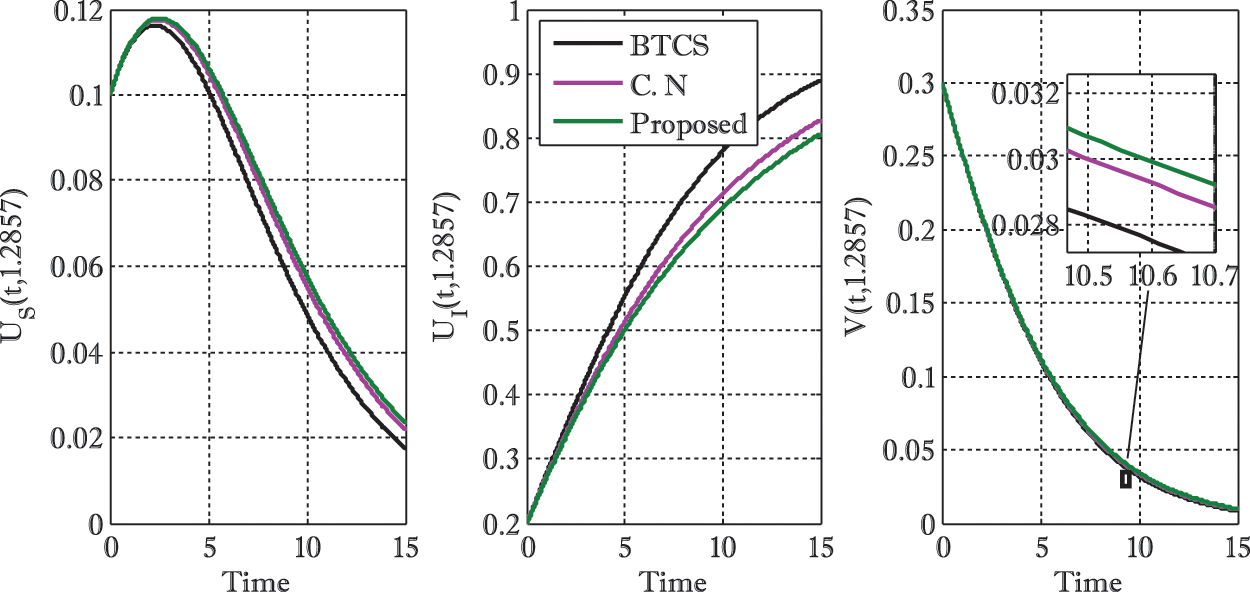Figure 4: Comparison of three schemes over time using a=0.1,b=0.2,f=0.1,α1=0.3,β=0.2,m1=0.1,α2=0.2,m2=0.1,c=0.1,g=0.1,n1=0.1,Nx=50,Nt=500,tf=15,L=7,d1=d2=d3=d4=0.4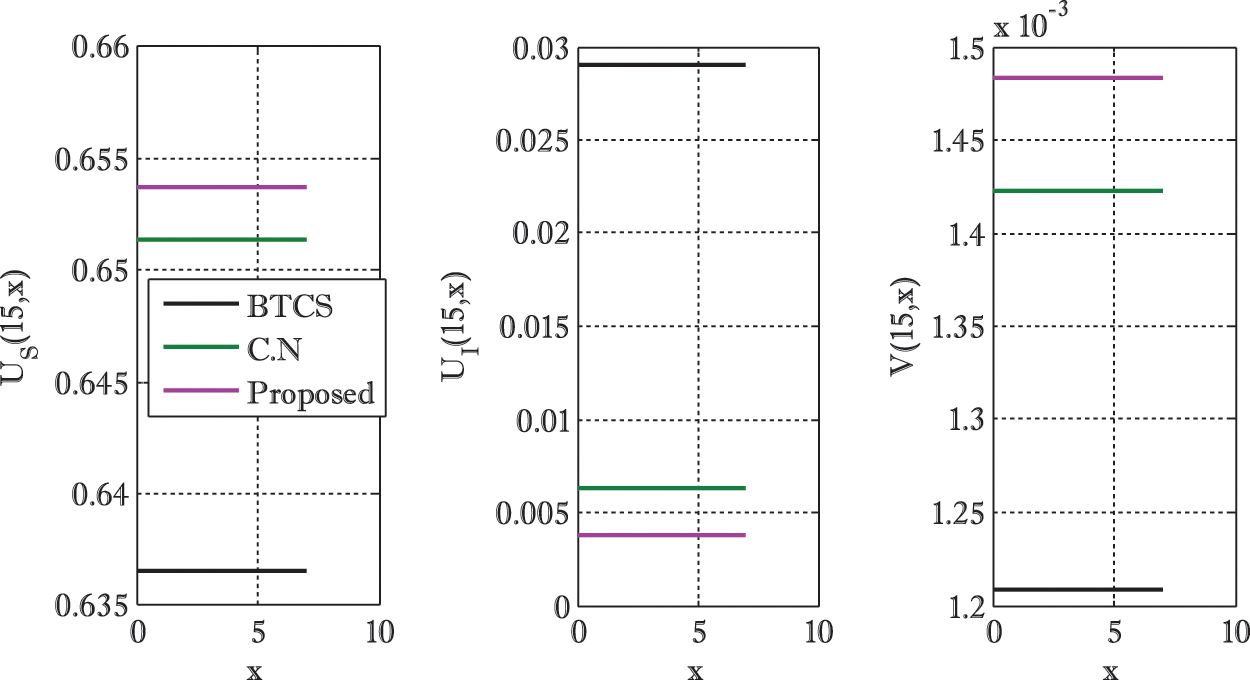Figure 5: Comparison of three schemes over space variable using a=0.1,b=0.01,f=0.2,α1=0.1,β=0.2,m1=0.3,α2=0.5,m2=0.3,c=0.3,g=0.1,n1=0.3,Nx=50,Nt=500,tf=15,L=7,d1=d2=d3=d4=0.4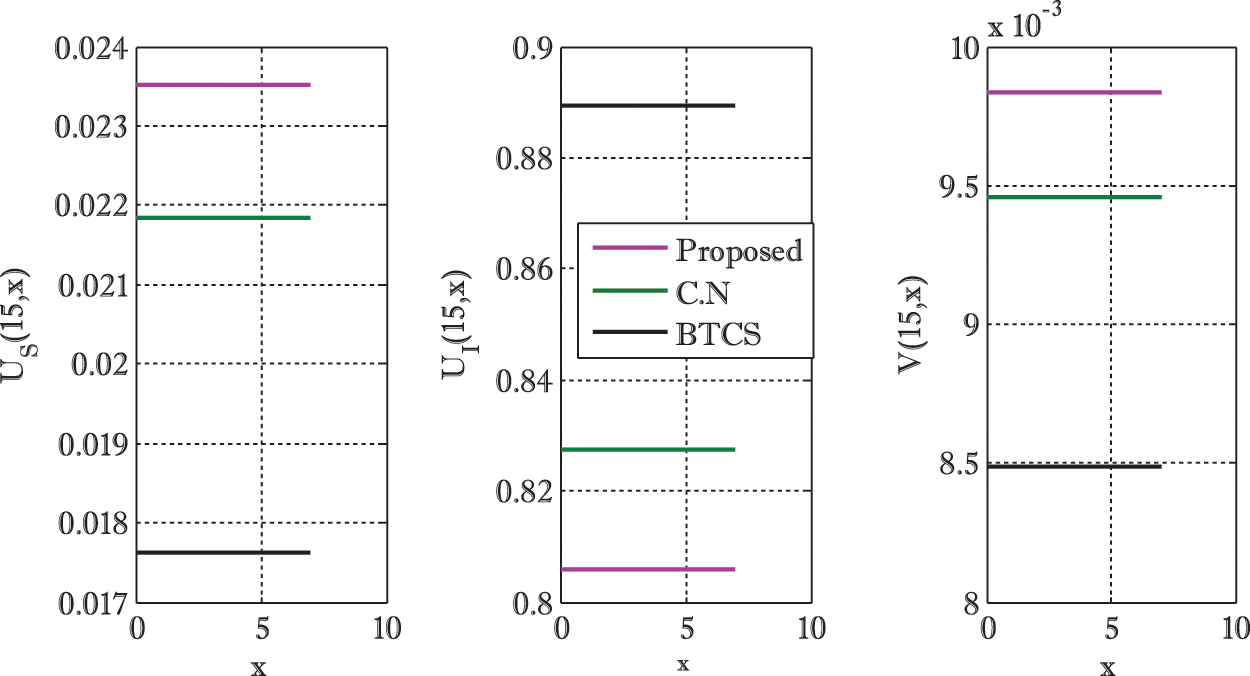Figure 6: Comparison of three schemes over space variable using a=0.1,b=0.2,f=0.1,α1=0.3,β=0.2,m1=0.1,α2=0.2,m2=0.1,c=0.1,g=0.1,n1=0.1,Nx=50,Nt=500,tf=15,L=7,d1=d2=d3=d4=0.4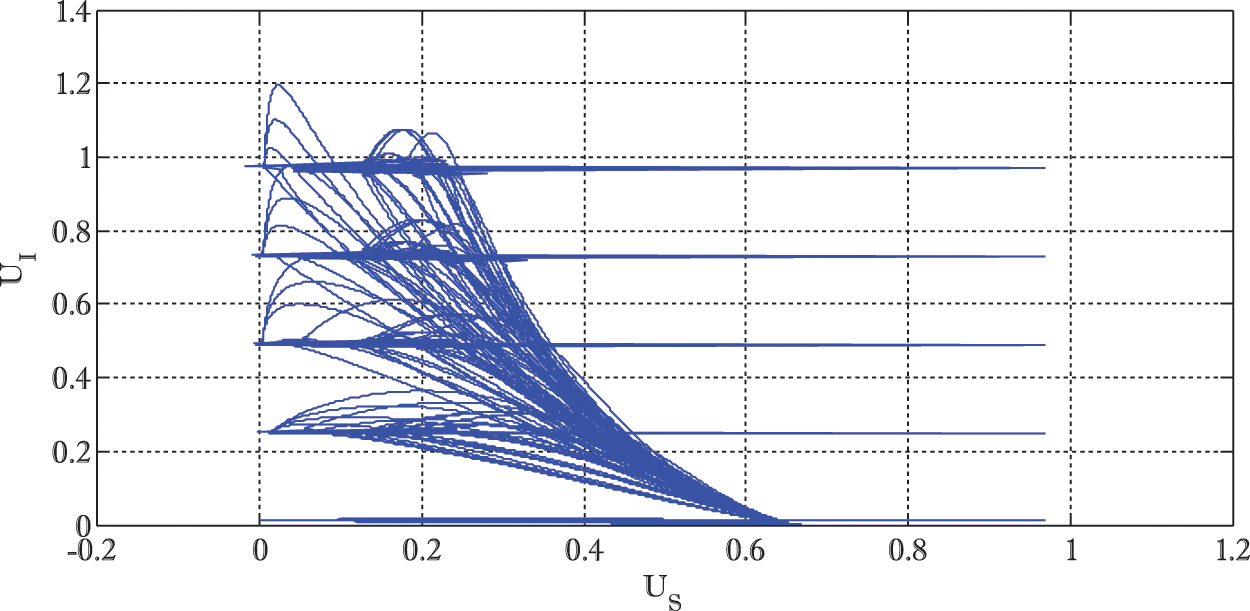Figure 7: Phase portrait of susceptible and infected predators US & UI using a=0.1,b=0.01,f=0.2,α1=0.1,β=0.2,m1=0.3,α2=0.5,m2=0.3,c=0.3,g=0.1,n1=0.3,Nt=500,tf=15Figure 8: Phase portrait of infected predators and prey population density UI & V using a=0.1,b=0.01,f=0.2,α1=0.1,β=0.2,m1=0.3,α2=0.5,m2=0.3,c=0.3,g=0.1,n1=0.3,Nt=500,tf=15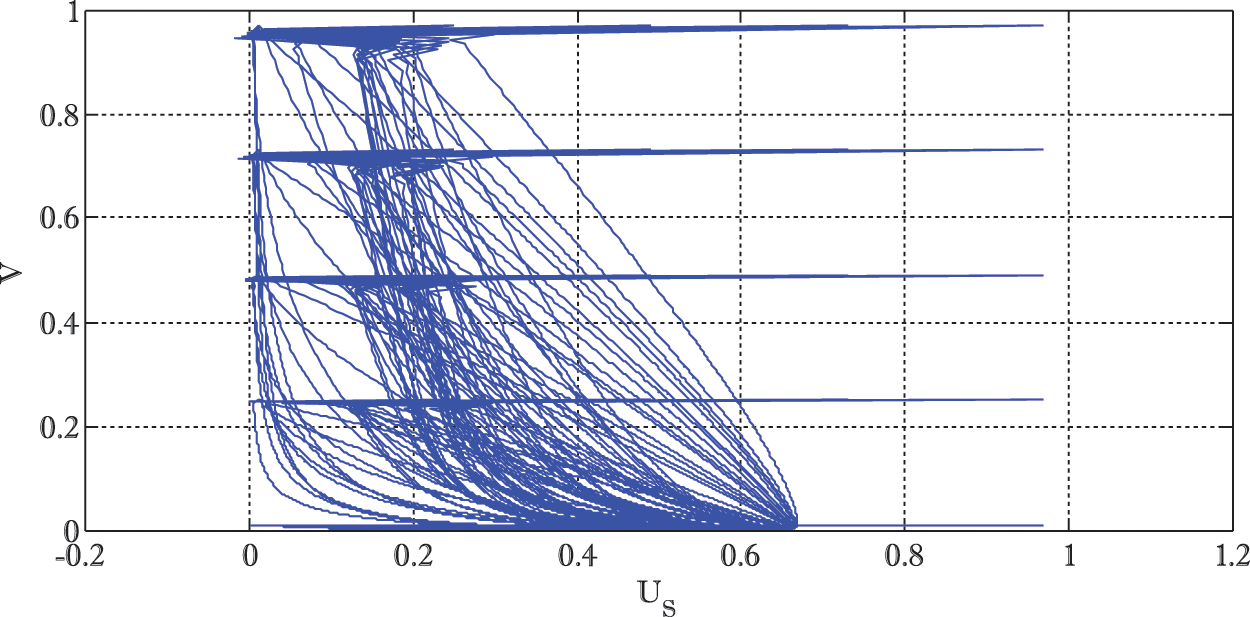Figure 9: Phase portrait of susceptible predators and prey population density US & V using a=0.1,b=0.01,f=0.2,α1=0.1,β=0.2,m1=0.3,α2=0.5,m2=0.3,c=0.3,g=0.1,n1=0.3,Nt=500,tf=15

First six Figs. 16 compare the presented numerical scheme with existing first and second-order schemes. The comparison is made in faster convergence to get the converged solution. If the scheme stops earlier than other schemes, it consumes fewer iterations than the other schemes. The faster convergence is obtained due to the higher order of accuracy of the proposed scheme than existing schemes. Therefore, the targeted solution is obtained by consuming fewer numbers of iterations. Also, the comparison is made by finding solutions of the considered model with diffusion effects over time and space coordinates. Solutions to the presented model are obtained by applying three different schemes. The change in the three solutions can be seen in Figs. 36. Again, the effect of using a higher order scheme can be observed in these figures. Since only straight lines can be seen for the solutions of the considered model over space coordinate x, this does not mean that solution is always just a straight line. The more detailed behavior of the solution over space coordinate x can be seen when it is formed only for a single scheme in one plot. So, in this manner, the solution in the form of some curves can be seen.

The solution curves are straight, showing that a very small change in the solution is found when it is drawn on the space coordinate x. So, the effect of adding diffusion term in the ODEs model produces a small change in the solution over spatial variable x. The rest of Figs. 79 shows the phase portrait that shows relationships between the predator and prey. Every curve in these Figs. 79 shows the relationship between a mentioned category of predator and prey corresponding to chosen initial conditions. An increase or decrease in any predator or prey categories can be seen over the increase in the corresponding drawn categories.

8  Conclusion

In the present article, we have proposed a dynamical non-linear eco-epidemiological population model considering two species, the predators (susceptible and infected) and prey, assuming the dependence of the predator classes (susceptible and infected) on the same food resource f. Additionally, a fatal transmissible disease was considered in the predator species, which only spread non vertically in the predator species. The equilibrium points of the model were calculated and analyzed for stability. The model was further studied by incorporating diffusion into the system. The stability of coexistence equilibrium points was proved theoretically by constructing the Lyapunov function. We have proved that the coexisting equilibrium point (US,UI,V) is stable for the original and self-diffusive system. We have constructed a numerical scheme that is successfully applied for solving the eco-epidemiological mathematical model. The third-order accuracy was obtained in the construction of the scheme, and it provided faster convergence when it was compared with first and second-order accurate (in-time) schemes.

Acknowledgement: We thank anonymous referees always warmly. The third and fifth authors wish to express their gratitude to Prince Sultan University for facilitating the publication of this article through the research lab Theoretical and Applied Sciences Lab.

Future Directions: In the present work, we have formulated a model using first-order ordinary differential equations and analyzed the stability of coexisting fixed points. The present work can be investigated using a stochastic approach in the future. Moreover, a Non-Standard Finite Difference Scheme can be used for numerical simulation and authentication of the model. Mathematical analysis of the model can be extended to further investigate bifurcation in the model.

Funding Statement: The authors would like to acknowledge the support of Prince Sultan University for paying the Article Processing Charges (APC) of this publication.

Conflicts of Interest: The authors declare that they have no conflicts of interest to report regarding the present study.

References

1.  1.  Volterra, V. (1926). Variazioni e fluttuazioni del numero d’individui in specie animali conviventi. Memoria della Reale Accademia Nazionale dei Lincei, 2, 31–113.
2.  2.  Lotka, A. (1925). Elements of physical biology. Baltimore: Williams and Wilkins.
3.  3.  Kermack, W. O., McKendrick, A. G. (1927). A contribution to the mathematical theory of epidemics. Proceedings of the Royal Society A, 115(5), 700–721. DOI 10.1098/rspa.1927.0118.
4.  4.  Maiti, A., Jana, M. M., Samanta, G. P. (2007). Deterministic and stochastic analysis of a ratio-dependent predator-prey system with delay. Nonlinear Analysis: Modelling and Control, 12(3), 383–398. DOI 10.15388/NA.2007.12.3.14700.
5.  5.  Wang, F., Kuang, Y., Ding, C., Zhang, S. (2013). Stability and bifurcation of a stage-structured predator-prey model with both discrete and distributed delays. Chaos, Solitons & Fractals, 46(4), 19–27. DOI 10.1016/j.chaos.2012.10.003.
6.  6.  Haque, M., Jin, Z., Venturino, E. (2009). An eco-epidemiological predator-prey model with standard disease incidence. Mathematical Methods in the Applied Sciences, 32(7), 875–898. DOI 10.1002/mma.1071.
7.  7.  Haque, M., Venturino, E. (2006). The role of transmissible diseases in Holling-Tanner predator-prey model. Theoretical Population Biology, 70(3), 273–288. DOI 10.1016/j.tpb.2006.06.007.
8.  8.  Yi-min, L., Yan, Z. (2009). The control and the reconfigurable control for prey-predator ecosystem with time delay. Applied Mathematical Modelling, 33(1), 148–160. DOI 10.1016/j.apm.2007.10.024.
9.  9.  Baleanu, D., Sajjadi, S. S., Jajarmi, A., Defterli, Ö. (2021). On a nonlinear dynamical system with both chaotic and nonchaotic behaviors: A new fractional analysis and control. Advances in Difference Equations, 2021(1), 1–17. DOI 10.1186/s13662-021-03393-x.
10. 10. Baleanu, D., Sajjadi, S. S., Asad, J. H., Jajarmi, A., Estiri, E. (2021). Hyperchaotic behaviors, optimal control, and synchronization of a nonautonomous cardiac conduction system. Advances in Difference Equations, 2021(1), 1–24. DOI 10.1186/s13662-021-03320-0.
11. 11. Baleanu, D., Sajjadi, S. S., Jajarmi, A. M. I. N., Defterli, O. Z. L. E. M., Asad, J. H. et al. (2021). The fractional dynamics of a linear triatomic molecule. Romanian Reports in Physics, 73(1), 1–13.
12. 12. Naji, R. K., Mustafa, A. N. (2012). The dynamics of an eco-epidemiological model with non-linear incidence rate. Journal of Applied Mathematics, 2012, 24. DOI 10.1155/2012/852631.
13. 13. Jang, S. R. J., Baglama, J. (2009). Continuous-time predator-prey models with parasites. Journal of Biological Dynamics, 3(1), 87–98. DOI 10.1080/17513750802283253.
14. 14. Sinha, S., Misra, O. P., Dhar, J. (2010). Modelling a predator-prey system with infected prey in polluted environment. Applied Mathematical Modelling, 34(7), 1861–1872. DOI 10.1016/j.apm.2009.10.003.
15. 15. Jana, S., Kar, T. K. (2013). Modeling and analysis of a prey-predator system with disease in the prey. Chaos, Solitons & Fractals, 47(3), 42–53. DOI 10.1016/j.chaos.2012.12.002.
16. 16. Johri, A., Trivedi, N., Sisdiya, A., Singh, B., Jain, S. (2012). Study of a prey-predator model with diseased prey. International Journal of Contemporay Mathematical Sciences, 7(10), 489–498.
17. 17. Mukhopadhyaya, B., Bhattacharyya, B. (2005). Dynamics of a delay-diffusion prey-predator model with disease in the prey. Journal of Applied Mathematics and Computing, 17(2), 361–377. DOI 10.1007/BF02936062.
18. 18. Greenhalgh, D., Haque, M. (2007). A predator-prey model with disease in the prey species only. Mathematical Methods in Applied Sciences, 30(8), 911–929. DOI 10.1002/(ISSN)1099-1476.
19. 19. Hu, G. P., Li, X. L. (2012). Stability and Hopf bifurcation for a delayed predator-prey model with disease in the prey. Chaos, Solitons & Fractals, 45(3), 229–237. DOI 10.1016/j.chaos.2011.11.011.
20. 20. Chattopadhyay, J., Arino, O. (1999). A predator-prey model with disease in prey. Nonlinear Analysis: Theory, Methods and Applications, 36(6), 747–766. DOI 10.1016/S0362-546X(98)00126-6.
21. 21. Baleanu, D., Zibaei, S., Namjoo, M., Jajarmi, A. (2021). A non-standard finite difference scheme for the modelling and non-identical synchronization of a novel fractional chaotic system. Advances in Difference Equations, 2021(1), 1–19, 308. DOI 10.1186/s13662-021-03454-1.
22. 22. Narayanamoorthy, S., Baleanu, D., Thangapandi, K., Perera, S. S. N. (2019). Analysis for fractional-order predator-prey model with uncertainty. IET Systems Biology, 13(6), 277–289. DOI 10.1049/iet-syb.2019.0055.
23. 23. Yusuf, A., Qureshi, S., Inc, M., Aliyu, A. I., Baleanu, D. et al. (2018). Two-strain epidemic model involving fractional derivative with Mittage-Leffer kernel. Chaos: An Interdisciplinary Journal of Nonlinear Science, 28(12), 123121. DOI 10.1063/1.5074084.
24. 24. Liu, M., Jin, Z., Haque, M. (2009). An impulsive predator-prey model with communicable disease in the prey species only. Nonlinear Analysis: Real World Applications, 10(5), 3098–3111. DOI 10.1016/j.nonrwa.2008.10.010.
25. 25. Naji, R. K., Hasan, K. A. (2012). The dynamics of prey-predator model with disease in the prey. Journal of Mathematics and Computer Science, 2(4), 1052–1072.
26. 26. Samanta, G. P. (2010). Analysis of a delay non-autonomous predator-prey system with disease in the prey. Nonlinear Analysis: Modelling and Control, 15(1), 97–108. DOI 10.15388/NA.2010.15.1.14367.
27. 27. Xiao, Y., Chen, L. (2001). Modeling and analysis of a predator-prey model with disease in the prey. Mathematical Biosciences, 171(1), 59–82. DOI 10.1016/S0025-5564(01)00049-9.
28. 28. Qureshi, S., Chang, M. M., Shaikh, A. A. (2021). Analysis of series RL and RC circuits with time-invariant source using truncated M, atangana beta and conformable derivatives. Journal of Ocean Engineering and Science, 6(3), 217–227. DOI 10.1016/j.joes.2020.11.006.
29. 29. Musa, S. S., Baba, I. A., Yusuf, A., Sulaiman, T. A., Aliyu, A. I. et al. (2021). Transmission dynamics of SARS-Cov-2: A modeling analysis with high and moderate risk population. Results in Physics, 26(102), 104290. DOI 10.1016/j.rinp.2021.104290.
30. 30. Liu, P., Din, A., Huang, L., Yusuf, A. (2021). Stochastic optimal control analysis for the hepatitis B epidemic model. Result in Physics, 26(2), 104372. DOI 10.1016/j.rinp.2021.104372.
31. 31. Hilker, F. M., Schmitz, K. (2008). Disease-induced stabilization of predator prey oscillations. Journal of Theoretical Biology, 255(3), 299–306. DOI 10.1016/j.jtbi.2008.08.018.
32. 32. Haque, M. (2010). A predator-prey model with disease in the predator. Nonlinear Analysis: Real World Applications, 11(4), 2224–2236. DOI 10.1016/j.nonrwa.2009.06.012.
33. 33. Pal, P. J., Haque, M., Mandal, P. K. (2003). Dynamics of a predator prey model with disease in the predator. Mathematical Methods in the Applied Scinces, 37(16), 2429–2450. DOI 10.1002/mma.2988.
34. 34. Owolabi, K. M., Karaagac, B., Baleanu, D. (2021). Pattern formation in superdiffusion predator-prey-like problems with integer-and noninteger-order derivatives. Mathematical Methods in the Applied Sciences, 44(5), 4018–4036. DOI 10.1002/mma.7007.
35. 35. Han, L., Zhien, M. A. (2001). Four predator prey models with infectious diseases. Mathematical and Computer Modelling, 34(7–8), 849–858. DOI 10.1016/S0895-7177(01)00104-2.
36. 36. Peter, O. J., Qureshi, S., Yusuf, A., Al-Shomrani, M., Idowu, A. A. (2021). A new mathematical model of COVID-19 using real data from Pakistan. Results in Physics, 24(2), 104098. DOI 10.1016/j.rinp.2021.104098.
37. 37. Qureshi, S. (2021). Fox H-functions as exact solutions for Caputo type mass spring damper system under Sumudu transform. Journal of Applied Mathematics and Computational Mechanics, 20(1), 83–89. DOI 10.17512/jamcm.2021.1.08.
38. 38. Hsieh, Y. H., Hsiao, C. K. (2008). Predator-prey model with disease infection in both populations. Mathematical Medicine and Biology, 25(3), 247–266. DOI 10.1093/imammb/dqn017.
39. 39. Nawaz, Y., Arif, M. S. (2021). Modified class of explicit and enhanced stability region schemes: Application to mixed convection flow in a square cavity with a convective wall. International Journal for Numerical Methods in Fluids, 93(6), 1759–1787. DOI 10.1002/fld.4951.
40. 40. Nawaz, Y., Arif, M. S. (2021). A new class of a-stable numerical techniques for ordinary differential equations: Application to boundary-layer flow. Thermal Sciences, 25(3A), 1665–1675. DOI 10.2298/TSCI190926097N.
41. 41. Nawaz, Y., Arif, M. S., Nazeer, A. (2021). A class of second-order schemes with application to chemically reactive radiative natural convection flow in a rectangular enclosure. International Journal of Numerical Methods in Fluids, 93(11), 3161–3186. DOI 10.1002/fld.5027.
42. 42. Nawaz, Y., Arif, M. S., Bibi, M., Abbasi, J. N., Javed, U. et al. (2020). A finite difference method and effective modification of gradient descent optimization algorithm for MHD fluid flow over a linearly stretching surface. Computer, Materials & Continua, 62(2), 657–677. DOI 10.32604/cmc.2020.08584.
43. 43. Nawaz, Y., Arif, M. S., Shatanawi, W., Nazeer, A. (2021). An explicit fourth-order compact numerical scheme for heat transfer of boundary layer flow. Energies, 14(12), 3396. DOI 10.3390/en14123396.
44. 44. Elettreby, M. F., Nabil, T., Khawagi, A. (2020). Stability and bifurcation analysis of a discrete predator-prey model with mixed holling interaction. Computer Modeling in Engineering & Sciences, 122(3), 907–921. DOI 10.32604/cmes.2020.08664.
45. 45. Khater, M. M., Almohsen, B., Baleanu, D., Inc, M. (2020). Numerical simulations for the predator-prey model as a prototype of an excitable system. Numerical Methods for Partial Differential Equations, 2020(6), 1–20. DOI 10.1002/num.22708.
46. 46. Qureshi, S., Yusuf, A., Shaikh, A., Inc, M., Baleanu, D. (2019). Fractional modeling of blood ethanol concentration system with real data application. Chaos, 29(1), 013143. DOI 10.1063/1.5082907.
47. 47. Fang, L., Wang, J. (2016). The global stability and pattern formations of a predator prey system with consuming resource. Applied Mathematics Letters, 58(2), 49–55. DOI 10.1016/j.aml.2016.01.020.This work is licensed under a Creative Commons Attribution 4.0 International License, which permits unrestricted use, distribution, and reproduction in any medium, provided the original work is properly cited.